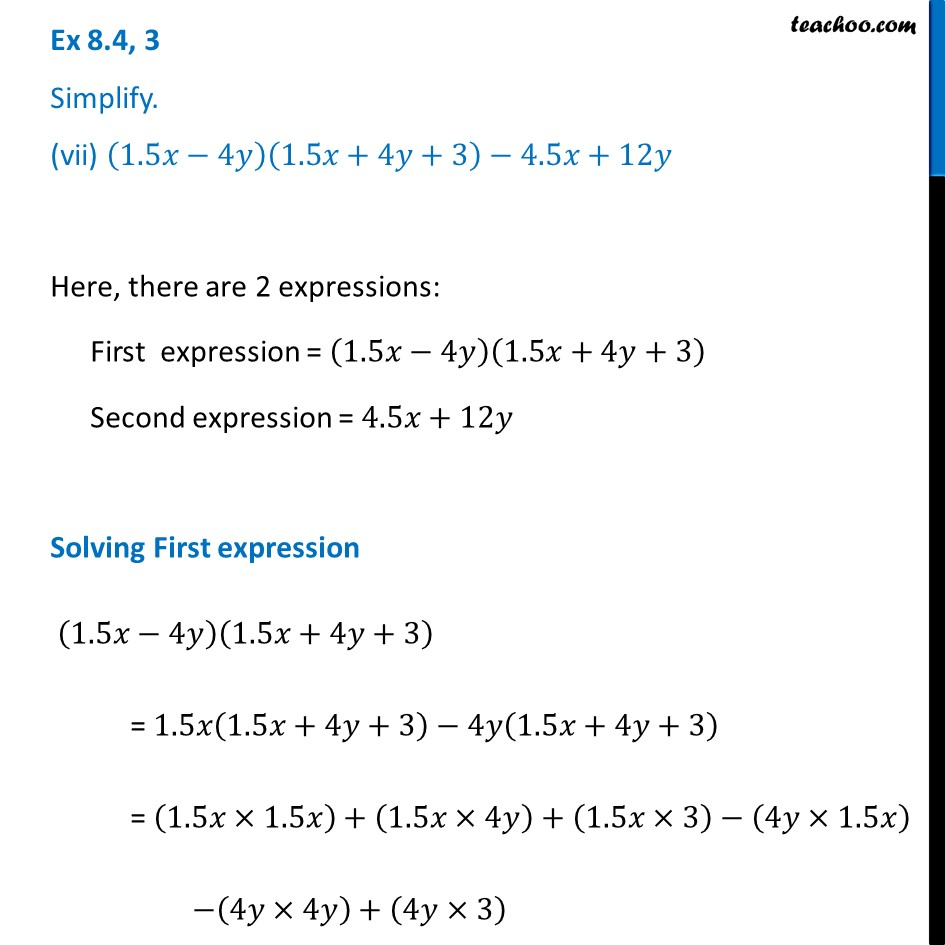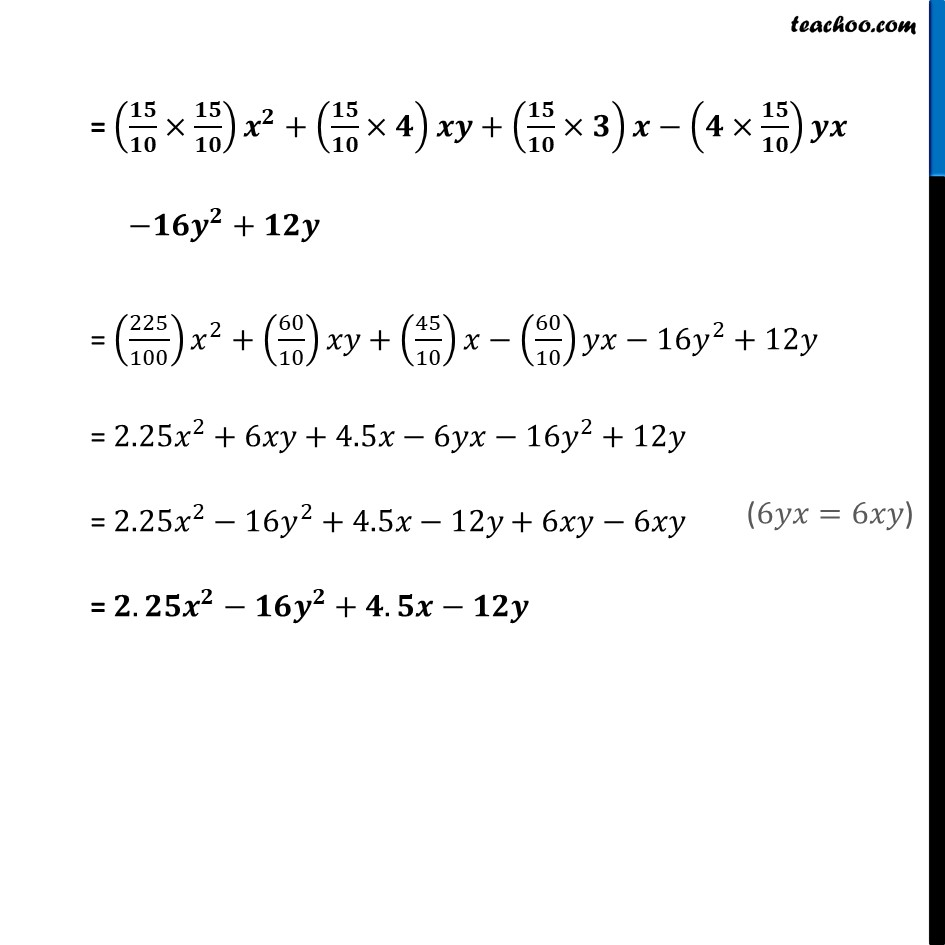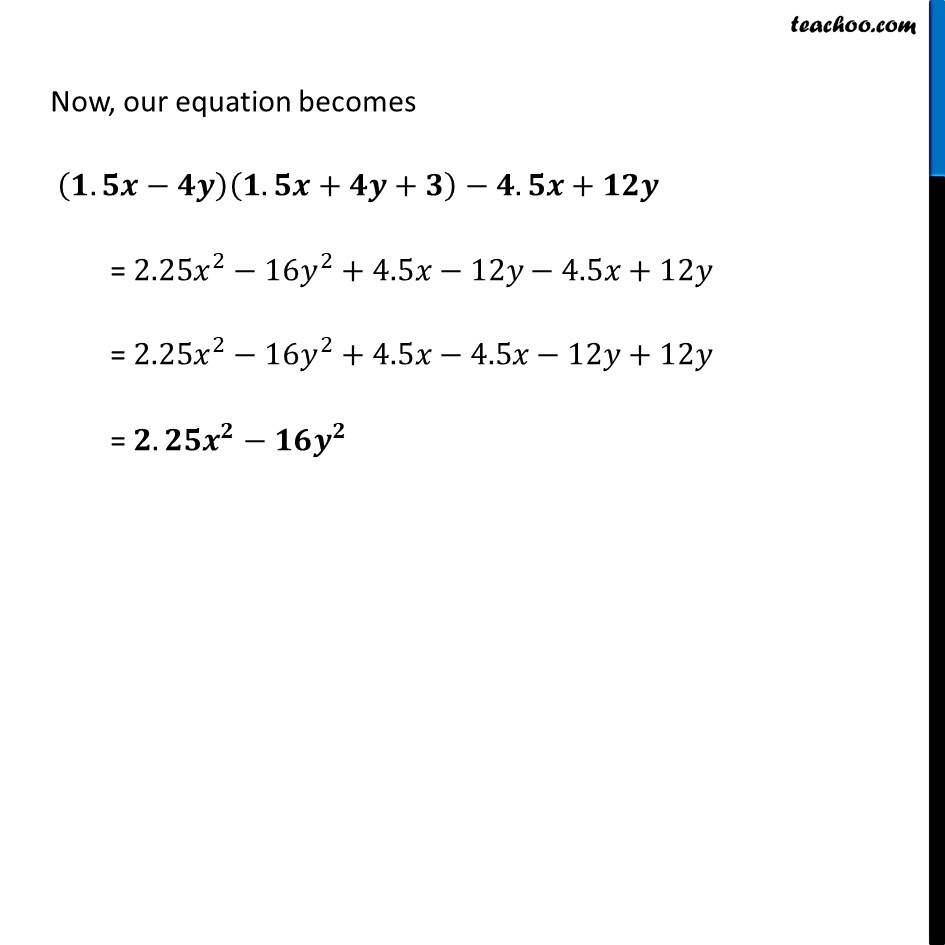Ex 8.4

Chapter 8 Class 8 Algebraic Expressions and Identities
Serial order wiseLearn in your speed, with individual attention - Teachoo Maths 1-on-1 Class

### Transcript

Ex 8.4, 3 Simplify. (vii) (1.5𝑥−4𝑦)(1.5𝑥+4𝑦+3)−4.5𝑥+12𝑦Here, there are 2 expressions: First expression = (1.5𝑥−4𝑦)(1.5𝑥+4𝑦+3) Second expression = 4.5𝑥+12𝑦 Solving First expression (1.5𝑥−4𝑦)(1.5𝑥+4𝑦+3) = 1.5𝑥(1.5𝑥+4𝑦+3)−4𝑦(1.5𝑥+4𝑦+3) = (1.5𝑥×1.5𝑥)+(1.5𝑥×4𝑦)+(1.5𝑥×3)−(4𝑦×1.5𝑥) −(4𝑦×4𝑦)+(4𝑦×3) = (𝟏𝟓/𝟏𝟎×𝟏𝟓/𝟏𝟎) 𝒙^𝟐+(𝟏𝟓/𝟏𝟎×𝟒)𝒙𝒚+(𝟏𝟓/𝟏𝟎×𝟑)𝒙−(𝟒×𝟏𝟓/𝟏𝟎)𝒚𝒙 −𝟏𝟔𝒚^𝟐+𝟏𝟐𝒚 = (225/100) 𝑥^2+(60/10)𝑥𝑦+(45/10)𝑥−(60/10)𝑦𝑥−16𝑦^2+12𝑦 = 2.25𝑥^2+6𝑥𝑦+4.5𝑥−6𝑦𝑥−16𝑦^2+12𝑦 = 2.25𝑥^2−16𝑦^2+4.5𝑥−12𝑦+6𝑥𝑦−6𝑥𝑦 = 𝟐.𝟐𝟓𝒙^𝟐−𝟏𝟔𝒚^𝟐+𝟒.𝟓𝒙−𝟏𝟐𝒚 Now, our equation becomes (𝟏.𝟓𝒙−𝟒𝒚)(𝟏.𝟓𝒙+𝟒𝒚+𝟑)−𝟒.𝟓𝒙+𝟏𝟐𝒚 = 2.25𝑥^2−16𝑦^2+4.5𝑥−12𝑦−4.5𝑥+12𝑦 = 2.25𝑥^2−16𝑦^2+4.5𝑥−4.5𝑥−12𝑦+12𝑦 = 𝟐.𝟐𝟓𝒙^𝟐−𝟏𝟔𝒚^𝟐